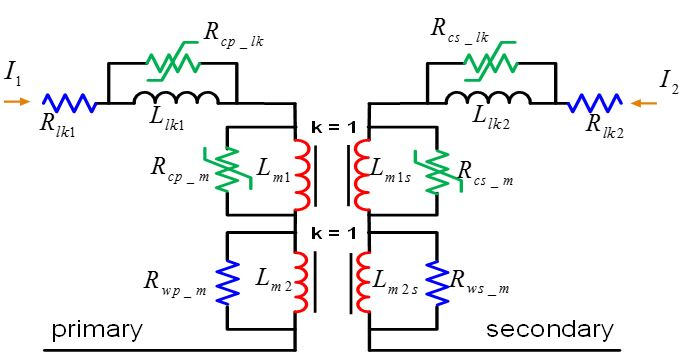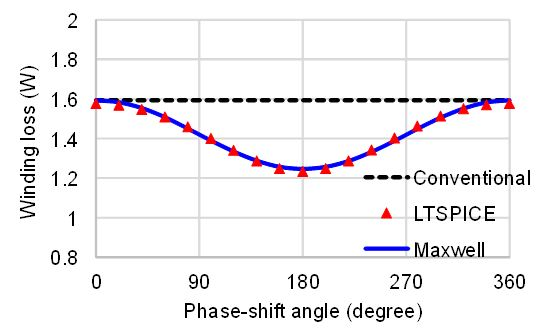LIBRARY

# Physics-Based Equivalent Circuit for Coupled Windings with Significant FringingFig. 1. Physics-based equivalent circuit for coupled windings
An equivalent circuit for coupled windings is developed for inductors with significant fringing effect. The equivalent circuit is derived from a physical model that captures the flux paths through a leakage inductor and two mutual inductors on the primary and secondary sides. Each side has a winding resistor in parallel with one mutual inductor to model winding loss with open circuit and phase-shift impact. Two time-varying resistors are employed to represent the core loss dynamically. The equivalent circuit is verified by both finite-element simulation (FES) and prototypes fabricated with a flexible circuit.

The equivalent circuit is shown in Fig. 1 with two winding resistors and two core loss resistors on each side. Rwp and Rws model the winding loss from coupling even if no net current is going through the winding. The core loss resistors are determined by core-loss calculation sub-circuit that dynamically predicts the core loss in the time domain.

Compared to the conventional equivalent circuit for coupled windings, the model herein is capable of predicting the winding loss when the phase shift between the primary and secondary currents is swept from 0 to 360 degrees.Fig. 2. Comparison of winding loss versus phase shift